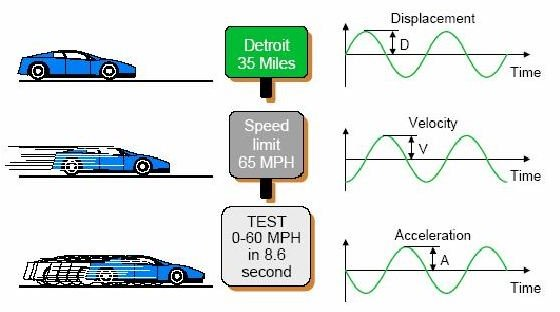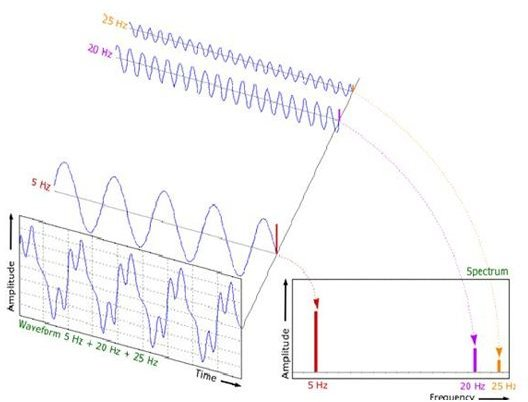# Terminology in Vibration: Includes Amplitude, Frequency, Time Domain & Other Vibration Terms

Terminologies in Vibration:

Let me just start with the basic terms before we get to the really tough ones.

1. Amplitude: This is the term that specifies the movement of the body. Well just think how we measure movement. It can be
1. Displacement – amount of movement from one point to another. E.g. I just walked 100 meters.
2. Velocity – the rate of movement, E.g. I moved the 100 meters in 10 seconds
3. Acceleration – The rate of change of velocity. E.g. The car has the capability to go from 0 mph to 100 mph in 8 Seconds.

These terms form the basis for the amplitude of vibration. Any measurement of vibration is normally denoted in the above three terms only. This also is incidentally the Y – Axis in any vibration related “graphs” as you call them. A small illustration below is given herewith to help you in understanding the terms better.

1. Frequency: While this is a common term in English, denoting how often something occurs, the same thing applies in vibration too. This denotes how frequently something occurs. For example, the full moon appears once in a month, certain features appear at regular intervals or they are made to appear at regular intervals based on their relative motion. We do have a small mathematical formula for rotating members to know their frequency, it is

Frequency,(Hz) = speed in rpm/60

The Hz denotes Hertz, the unit for frequency.

These two terms are the primary ones that everyone needs to know. But we need to know more about two important terminologies before we move on to look at the other terms. Vibration is frequently related with time. To be more specific we can say that vibration is more of a time-dependent data. This is why we need to know more about the two important terms before we proceed to the other terms. They are:

Time Domain: To say in a very simple way we can think of this as a graph with Time in the X – Axis and Amplitude in the Y – Axis. You can assume the amplitude to be for example the amount of height a body jumps due to vibration. You can also keep the unit as "mm". A good example of the visualizing Time Domain data would be the Sine Wave. This is the form in which we visualize or look at the vibration data with respect to time. But this tends to give lesser information on vibration. Let us discuss more on this in my later articles.

Frequency or Spectrum Domain: This is another dimension to the Time Domain data you see. Again visualize this as a graph with Frequency in the X – Axis and Amplitude in the Y – Axis. Just see the adjoining figure to clearly understand what Time Domain and Frequency Domain are.

Understanding these two domains is the real foundation for anyone who needs to pursue a career in the field of Vibration Engineering. We will look more into other terminologies in the second chapter of this article.